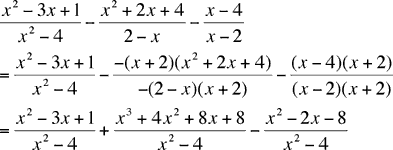Quandaries and Queries I am in 11th grade Honors Algebra II. We are currently learning about adding and subtracting rational expressions. I looked at the other questions that were similar to that of mine, but they weren't quite answering my question. I have been working on many problems to practice and see if I can come up with the correct answer, but I can't, I got like 2 out of every 7 right on my school homework. I get the easy ones, where when you have to find the LCD, it's just the other denominator. But, on others you have to really think about it. Those are hard. Here are some of the problems that I cannot get at all: (x2 - 3x + 1)/(x2 - 4) - (x2 + 2x +4)/(2 - x) - (x - 4) /(x - 2) The correct answer is: (x3 + 4x2 + 7x + 17)/(x2 - 4) -18 + 7 - 2 9xy 2x 3x2 The correct answer is: -12x + 21xy - 4y 6x²y I just couldn't get the right answers, if you could explain how to arrive at those answers, I would greatly appreciate it. Thank You so much, Thank you again, Shivani Hi Shivani , In the first problem the denominators are (x2 - 4), (2 - x) and (x - 2). The important fact is to see that (x2 - 4) can be factored as a difference of squares. Thus (x2 - 4) = (x - 2)(x + 2) Since the second and third denominators both divide this, you can take (x2 - 4) as a common denominator. [For the second denominator (x2 - 4) = -(2 - x)(x + 2).] Thus,Simplification gives you the correct answer. The second problem arrived in a garbled fashion but I can see the four denominators. They are 9xy, 2x, and 3x2. Hence a common denominator is 9xy2x = 18x2y Penny Go to Math Central